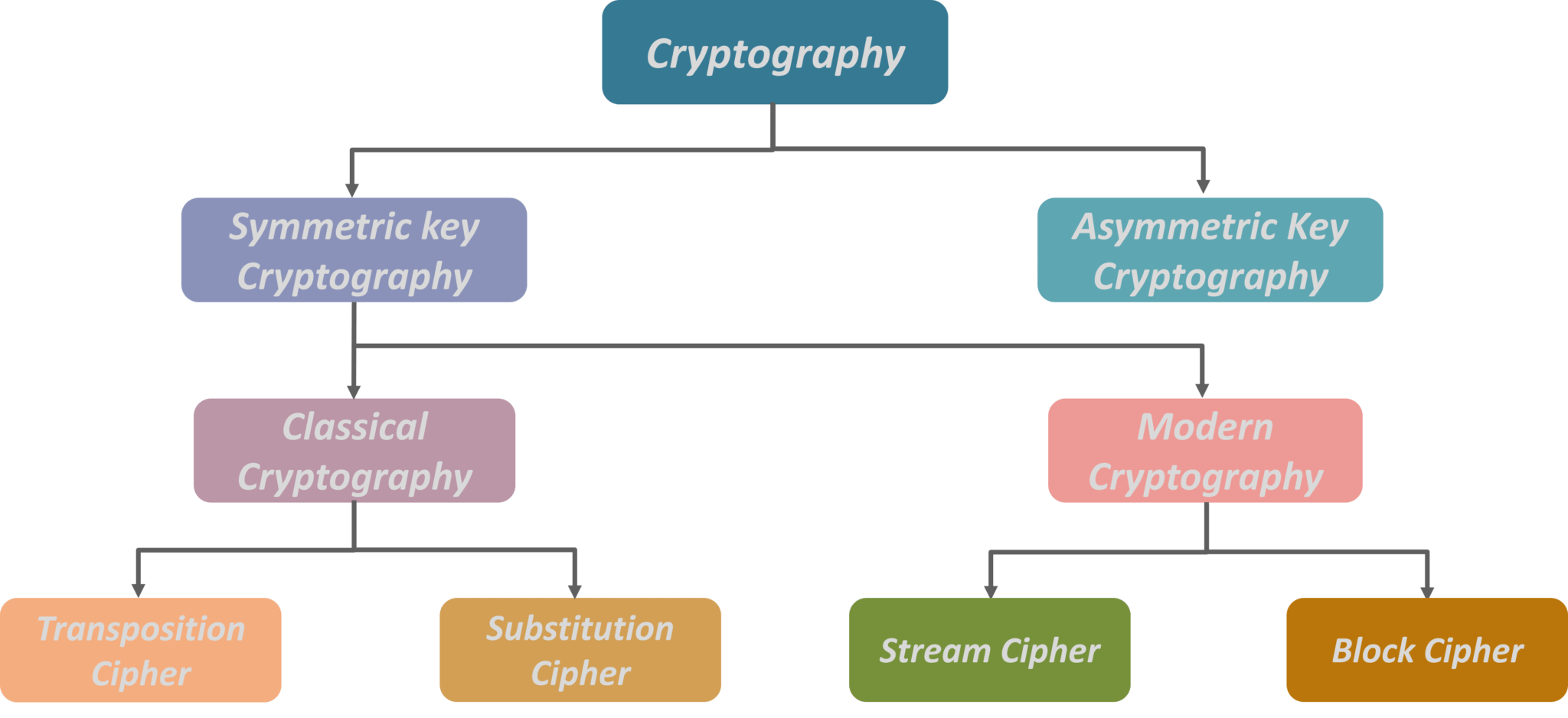# New Technology

Trending Technology Machine Learning, Artificial Intelligent, Block Chain, IoT, DevOps, Data Science

## Search This Blog

Introduction to Cryptography

Cryptography is the practice and study of hiding information. I is a branch of both mathematics and computer science, and is affiliated closely with information theory, computer security, and engineering.

Cryptography is used in applications present in technologically advanced societies. Ex: ATM cards, computer passwords, and electronic commerce etc.

Cryptography : The science of keeping messages secure

Cryptanalysis : The science of breaking the ciphertext without the key.

Cryptology : The branch of mathematics encompassing both cryptography and cryptanalysis.Encryption : A process of encoding a message, so that its meaning is not obvious. ( = encoding, enciphering)

Decryption : A process of decoding an encrypted message back into its original form. ( = decoding, deciphering)RSA Algorithm :

Developed by Rivest, Shamir and Adleman at MIT in 1978. Hence the name RSA

Based on the difficulty of factoring the product of two primes, p and q.

Let p x q = n;

Given n, it is extremely difficult to find p and q

An Example :

• Get p, q which are two large primes.
• Find N = p x q
• Find Φ(N) = (p-1) x (q-1)
• Find two integers d and e such that : ( d x e) mod ( Φ(N) = 1.
• e is the encryption key.
• d becomes the decryption key.

Public Key Cryptography - Secrecy

A send Message M to B, by applying B's public_key, Pub_B

Public keys are available to everyone

C = Pub_B (M)

B recovers the message by applying his private key

Pri_B (C) = Pri_B (Pub_B(M)) = M

Public Key Cryptography - Signature

A sends Message M to B, by applying A's private key, Pri_A

C = Pri_A (M)

B recovers the message by applying A's public key. (Public keys are available to everyone)

Pub_A (C) = Pub_A (Pri_A(M)) = M

Note that since the ciphertext has been obtained by applying A's Private_key. it amounts to his Digital Signature.

Also, since A's Private_Key has been applied to generate the ciphertext he cannot deny later that he send the ciphertext.